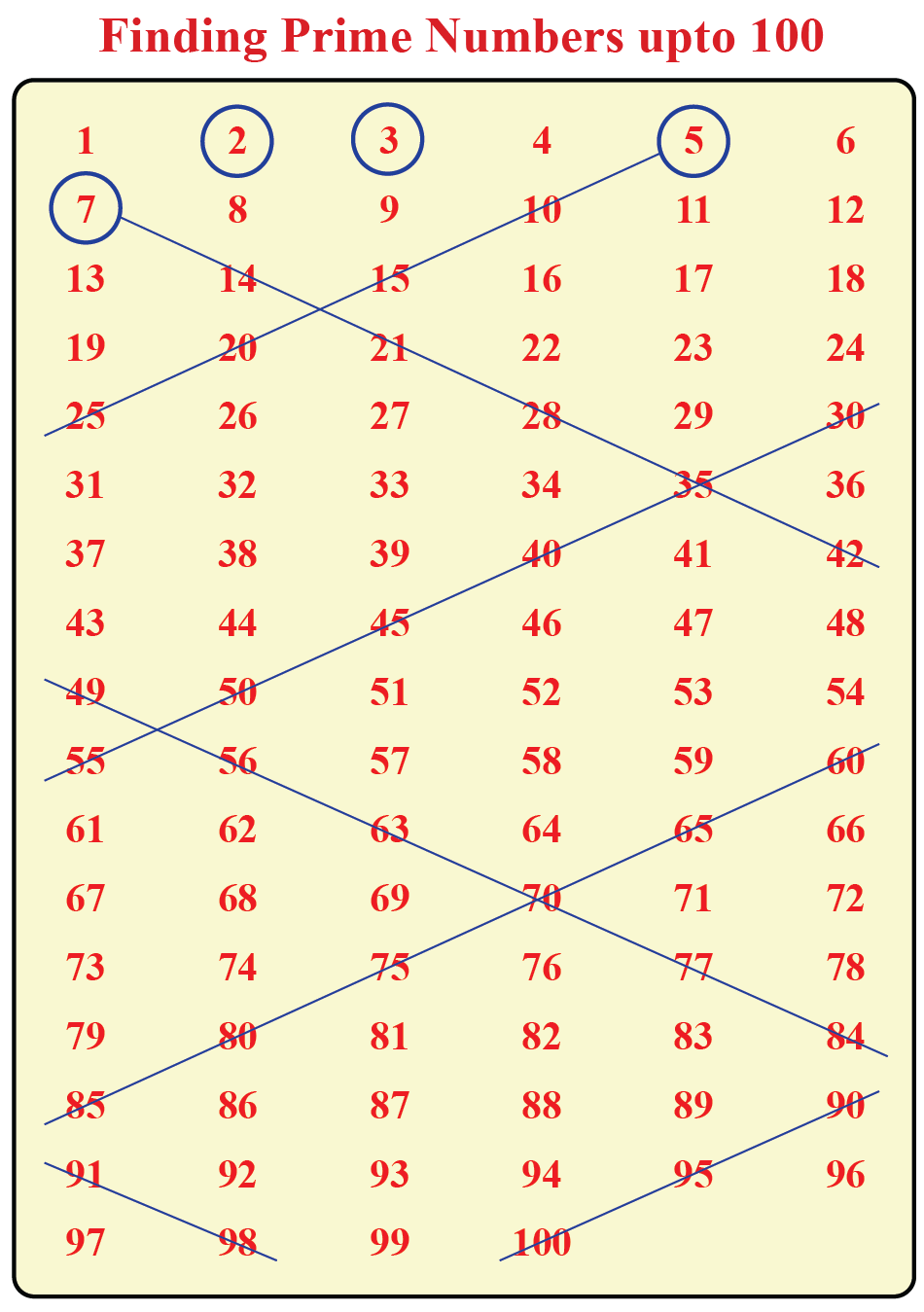# Prime Numbers and Euclids Proof

Prime Numbers and Euclids Proof

Prime numbers have been fascinating mathematicians for thousands of years.

A prime number is always greater than 1 and can only be divided by itself and 1 – no other number will divide into it. 2 is the first prime number (also the only even prime number), followed by 3, 5, 7, and so on.

Non-prime numbers are defined as composite numbers.

Prime numbers are distributed randomly throughout all the other numbers. Also, there is no simple and quick way to find a specific (new) prime number. As a result, large prime numbers are used in encryption to make the online platform protected for safe communication and financial transaction.

Did you know the number of primes is infinite? Explore Euclid’s Theorem of infinite primes with proof, solved examples and interactive questions, the Cuemath way.

## Lesson PLan

 1 2 What is Euclid’s Proof for Infinite Primes? 3 Solved Examples on Infinite Prime Numbers 4 Interactive Questions on Infinite Primes 5 Important Notes on Infinite Prime Numbers

## How Do You Identify Prime Numbers?

### Definition

In numbers, we have learnt that prime numbers have two factors - 1 and the number itself. To find a prime number, we need to find the numbers which consist of only two factors. This is possible by using a simple method, which is called prime factorization.

### Steps to Finding Prime Numbers Using Factorization

Step 1. Divide the number into factors

Step 2. Check the number of factors of that number. If the number of factors is more than 2 then it is composite.

Example: $$8$$ has four factors $$1,\, 2,\, 4,\, 8$$. So 8 and therefore is not prime
Step 3. All prime numbers greater than 3 can be represented by the formula $$6n+ 1$$ and $$6n -1) for n greater than equal to 1. Exception if the number ends with 5 or higher multiples of 7 such as 49, 77, 91 Examples: \(5=6\times 1-1$$

$$7=6\times 1+1$$

$$11=6\times 2-1$$

$$13=6\times 2-1$$

etc.### Steps to Identify A Large Number Is Prime Number

Lets us take a number $$1249$$

Step 1. If the number ends with $$0,\, 2,\, 4,\,5,\, 6\, and\, 8,$$ then it is not a prime number.

Step 2. Add the digits of your number if the number is divisible by $$3$$ then we can say that, it is not a prime number.
$$1249=1+2+4+9=16$$
Step 3. If the condition for steps 1 and 2 are not true, then find the square root of the number

$$\sqrt(1249)=35.3$$

Step 4. Divide the number by all prime numbers less than its square root value

Prime number less than $$35:\,\, 1,\,2,\,3,\,5,\,7,\,11,\,13,\,17,\,19,\,23,\,29,\,31$$

Step 5. If the number is divisible by any of the prime numbers less than its square root, it is not a prime number; otherwise, it is prime.

Hence, after following all steps we can say that $$1249$$ is a prime  number

## What Is Euclid’s Proof for Infinite Primes?

Euclid,  in 4th century B.C, pointed out that there has been an infinite number of primes. The concept of infinity is not known at that time. He said ”prime numbers are quite any fixed multitude of them” meaning if you imagine 100, there are more, and if you imagine a million there are still more.

### Euclid's Proof for an Infinite Number of Primes

let us assume that, there are a finite number of primes, $$n$$

the largest prime number is $$p_n$$.

Consider the number, $$N$$.  that is the product of these primes number plus one

By construction, N is not divisible by any of the $$p_i$$.

Hence it's either prime itself, or divisible by another prime greater than $$p_n$$ , contradicting the idea.

For example:

$$2 + 1 = 3$$, is prime
$$2\times 3 + 1 = 7$$, is prime
$$2\times 3 \times 5 + 1 = 31$$, is prime
$$2\times 3 \times 5\times 7 + 1 = 211$$, is prime
$$2\times 3 \times 5\times 7\times 11 + 1 = 2311$$, is prime
etc.

Thus, we can conclude this the number of primes is infinite.

## Solved Examples

 Example 1

Is $$233$$ a prime number?

Solution

If $$233$$ is a prime number, then it must not be divisible by a prime that is less than or equal to $$\sqrt{233}$$.$$\sqrt{213}$$ is between $$15$$ and $$16$$, so the largest prime number that is less than $$\sqrt{213}$$ is $$13$$. therefore we test $$2,\, 3,\, 5,\, 7,\, 11,\, and\, 13\,$$ for divisibility.
233 is not divisible by any of those numbers, so it must be prime

 $$233$$ is a prime number
 Example 2

<

Is $$1001$$ a prime number?

Solution

If $$1001$$ is a prime number, then it must not be divisible by a prime that is less than or equal to $$\sqrt{1001}$$.$$\sqrt{1001}$$ is between $$31$$ and $$32$$, so the largest prime number that is less than $$\sqrt{1001}$$ is $$31$$. It is therefore sufficient to test $$2,\, 3,\, 5,\, 7,\, 11,\,13,\,17,\,19,\, 23,\, 29,\, and\, 31$$ for divisibility.

$$1001=7 \times 11\times 13$$. $$1001$$ is divisible by $$7,\,11,\, and \, 13$$ so it is not a prime number

 $$1001$$ is not a prime number

## Interactive Questions

Here are a few activities for you to practice. Select/Type your answer and click the "Check Answer" button to see the result.

Arithmetic Integers